# Guass 's Law

## 4. Applications of Gauss's Law

### (A) Derivation of Coulumb's Law

• Coulumb's law can be derived from Gauss's law.
• Consider electric field of a single isolated positive charge of magnitude q as shown below in the figure.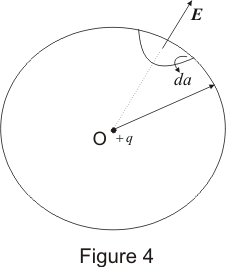• Field of a positive charge is in radially outward direction everywhere and magnitude of electric field intensity is same for all points at a distance r from the charge.
• We can assume Gaussian surface to be a sphere of radius r enclosing the charge q.
• From Gauss's law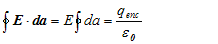since E is constant at all points on the surface therefore,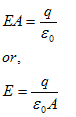surface area of the sphere is A=4πr2
thus,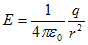• Now force acting on point charge q' at distance r from point charge q is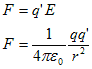This is nothing but the mathematical statement of Coulomb's law.

### (B) Electric field due to line charge

• Consider a long thin uniformly charged wire and we have to find the electric field intensity due to the wire at any point at perpandicular distance from the wire.
• If the wire is very long and we are at point far away from both its ends then field lines outside the wire are radial and would lie on a plane perpandicular to the wire.
• Electric field intensity have same magnitude at all points which are at same distance from the line charge.
• We can assume Gaussian surface to be a right circular cylinder of radius r and length l with its ends perpandicular to the wire as shown below in the figure.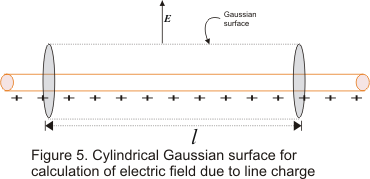• λ is the charge per unit length on the wire. Direction of E is perpendicular to the wire and components of E normal to end faces of cylinder makes no contribution to electric flux. Thus from Gauss's law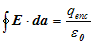• Now consider left hand side of Gauss's law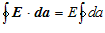Since at all points on the curved surface E is constant. Surface area of cylinder of radius r and length l is A=2πrl therefore,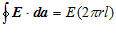• Charge enclosed in cylinder is q=linear charge density x length l of cylinder,
or, q=λl
From Gauss's law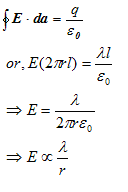Thus electric field intensity of a long positively charged wire does not depends on length of the wire but on the radial distance r of points from the wire.